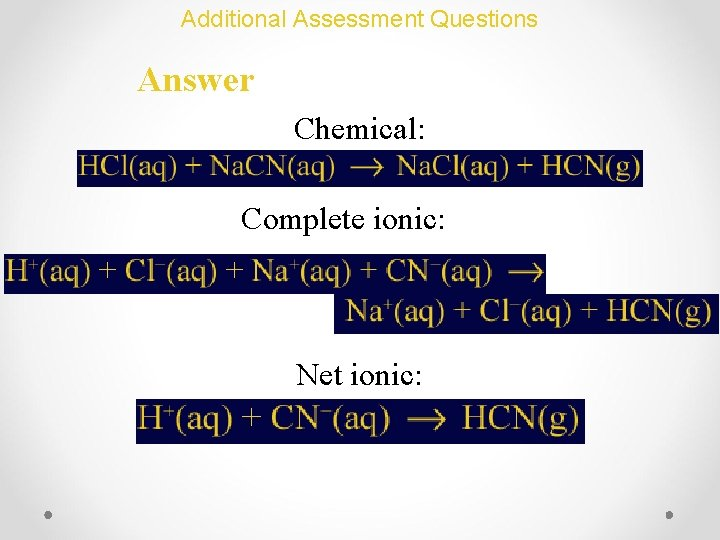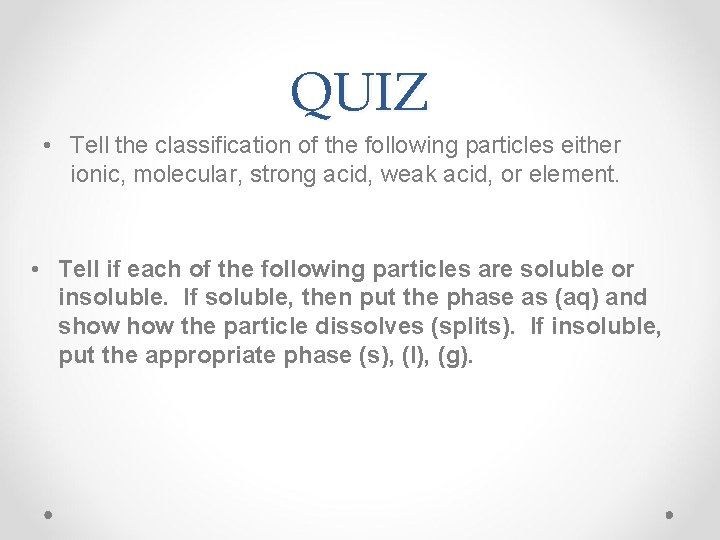• Slides: 55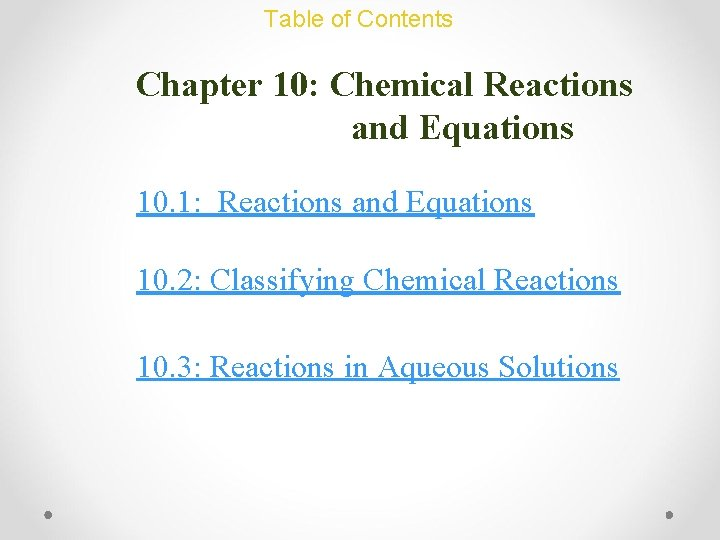Table of Contents Chapter 10: Chemical Reactions and Equations 10. 1: Reactions and Equations 10. 2: Classifying Chemical Reactions 10. 3: Reactions in Aqueous Solutions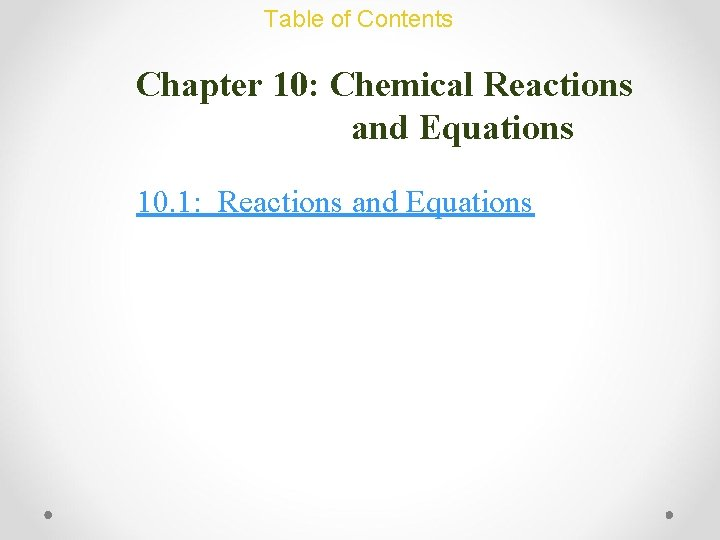Chemical Reactions and Equations: Basic Concepts Writing Chemical Equations • A substance that undergoes a reaction (a chemical change) is called a reactant. • Each new substance formed is called a product. • Reactants Products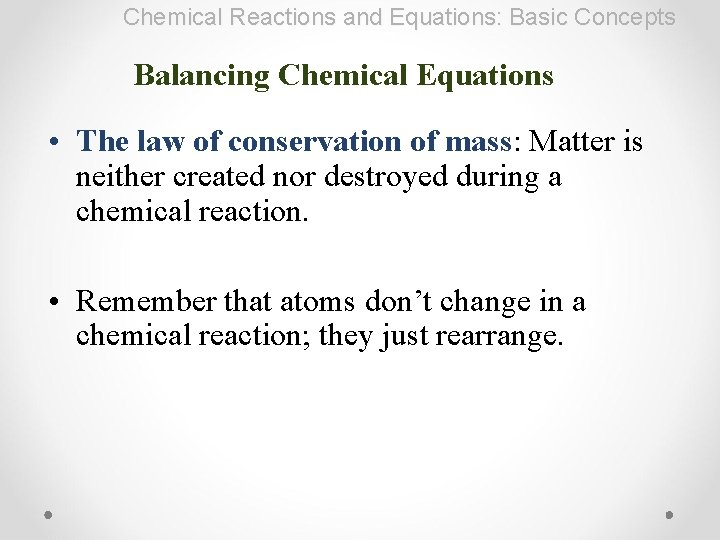Chemical Reactions and Equations: Basic Concepts Balancing Chemical Equations • The law of conservation of mass: Matter is neither created nor destroyed during a chemical reaction. • Remember that atoms don’t change in a chemical reaction; they just rearrange.Chemical Reactions and Equations: Basic Concepts Balancing Chemical Equations • Only one rule: Atoms on the left = Atoms on the rightChemical Reactions and Equations: Basic Concepts Balancing Chemical Equations • Skeleton Equations are unbalanced chemical equations. Not balanced!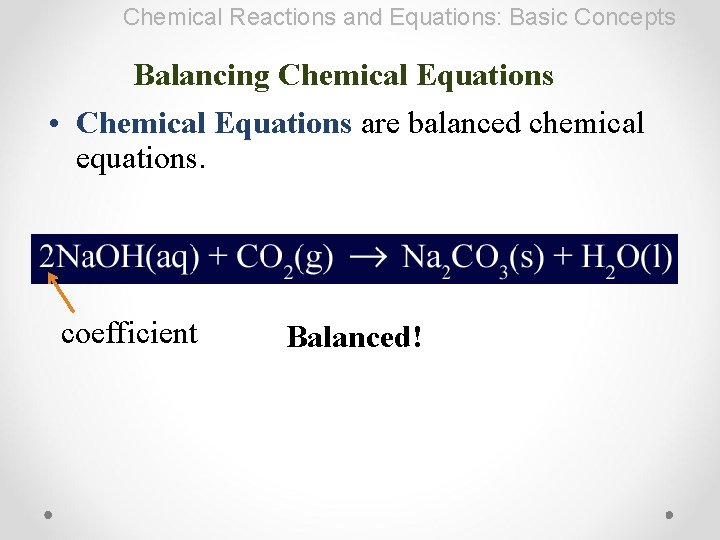Chemical Reactions and Equations: Basic Concepts Balancing Chemical Equations • Chemical Equations are balanced chemical equations. coefficient Balanced!Balancing an Equation Basic Hints: 1. Do easiest elements first. C 5 H 12(g) + 8 O 2(g) → 5 CO 2(g) + 6 H 2 O(l) 2. Save element coefficient for last. 4 PH 3 + 8 O 2(g) → P 4 O 10(s) + 6 H 2 O(g) 3. Save H & O for last, they often work themselves out. 2 Fe(OH)3 → Fe 2 O 3 + 3 H 2 O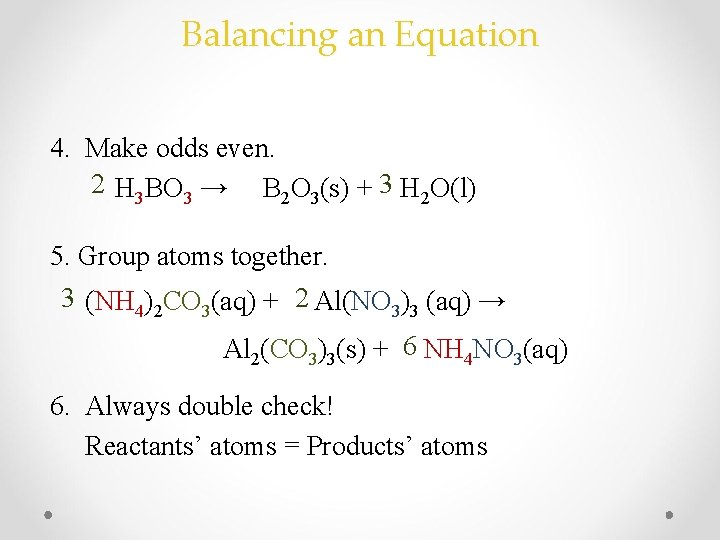Balancing an Equation 4. Make odds even. 2 H 3 BO 3 → B 2 O 3(s) + 3 H 2 O(l) 5. Group atoms together. 3 (NH 4)2 CO 3(aq) + 2 Al(NO 3)3 (aq) → Al 2(CO 3)3(s) + 6 NH 4 NO 3(aq) 6. Always double check! Reactants’ atoms = Products’ atomsTry it out! • Balancing Chemical Equations Worksheet – try 1 -5 now • The “Balancing Chemical Equations” side is due Thursday (tomorrow) • The “Balancing Equations Worksheet” side is for you if you need additional practice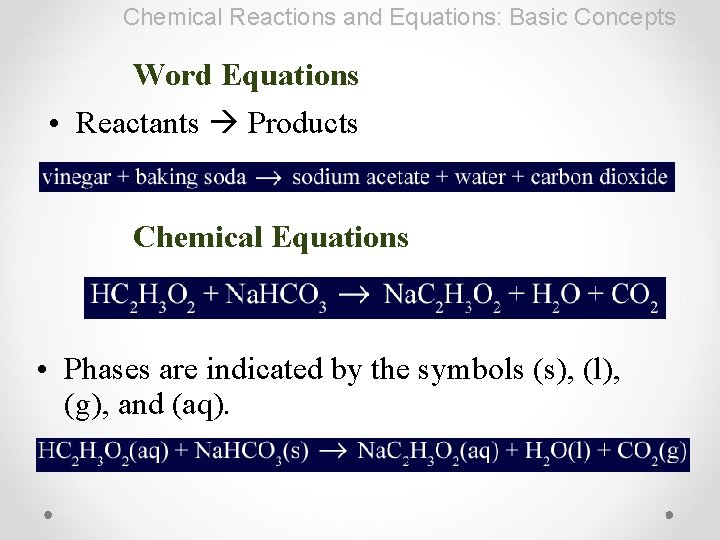Chemical Reactions and Equations: Basic Concepts Word Equations • Reactants Products Chemical Equations • Phases are indicated by the symbols (s), (l), (g), and (aq).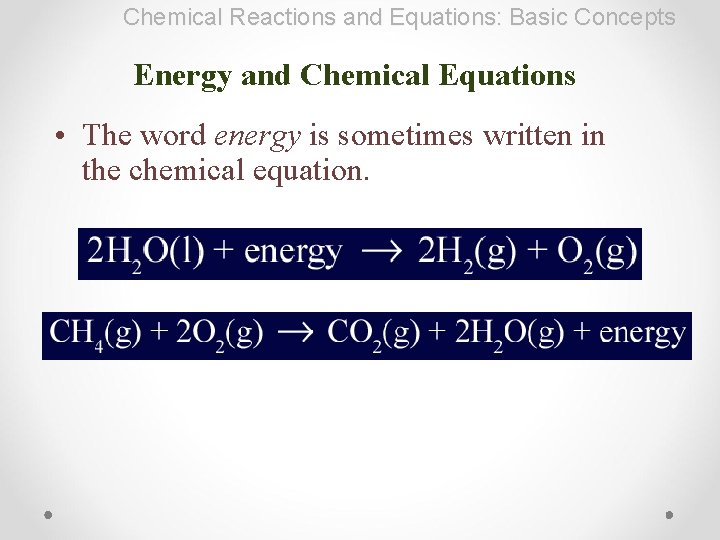Chemical Reactions and Equations: Basic Concepts Energy and Chemical Equations • The word energy is sometimes written in the chemical equation.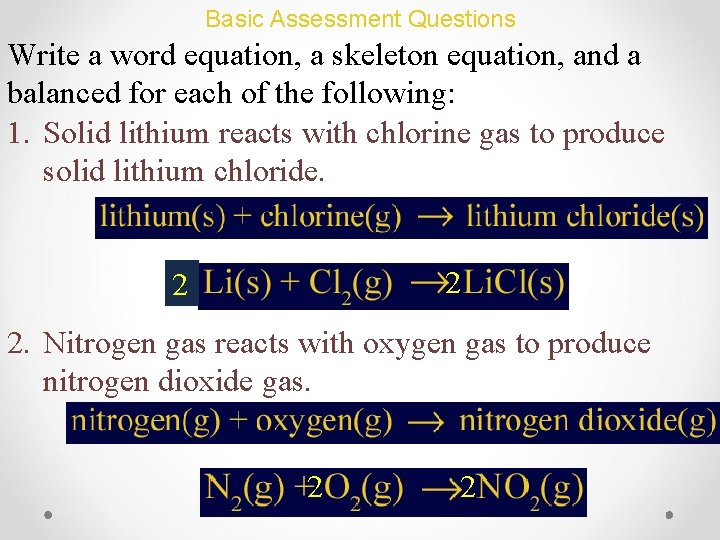Basic Assessment Questions Write a word equation, a skeleton equation, and a balanced for each of the following: 1. Solid lithium reacts with chlorine gas to produce solid lithium chloride. 2 2 2. Nitrogen gas reacts with oxygen gas to produce nitrogen dioxide gas. 2 2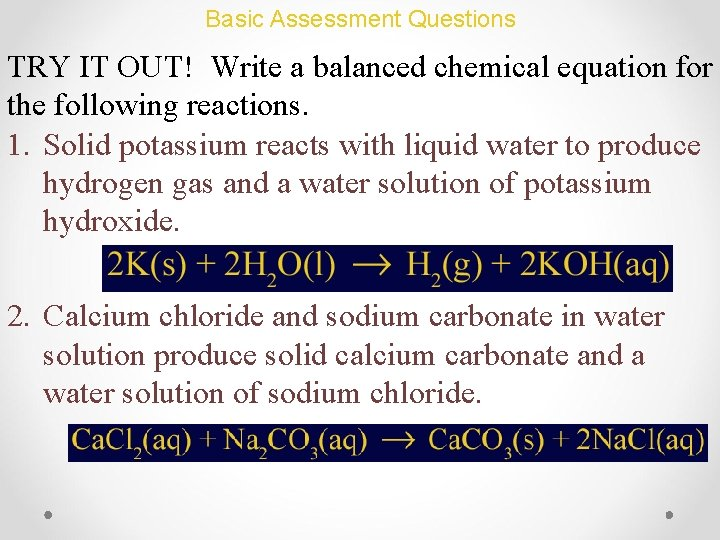Basic Assessment Questions TRY IT OUT! Write a balanced chemical equation for the following reactions. 1. Solid potassium reacts with liquid water to produce hydrogen gas and a water solution of potassium hydroxide. 2. Calcium chloride and sodium carbonate in water solution produce solid calcium carbonate and a water solution of sodium chloride.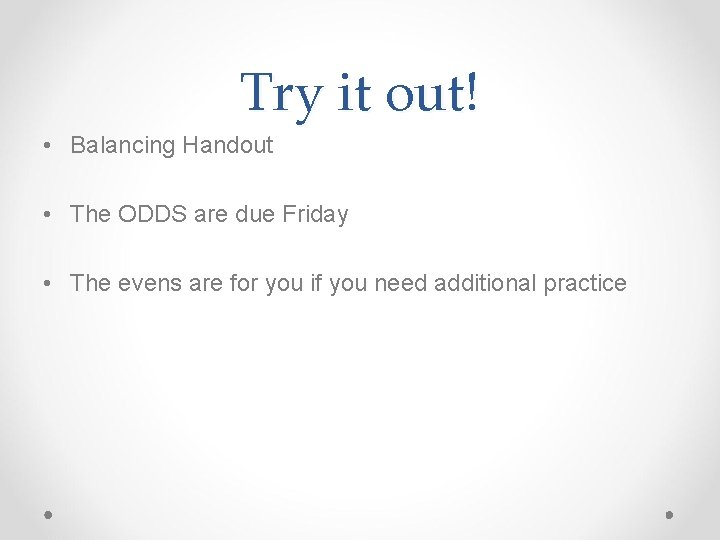Try it out! • Balancing Handout • The ODDS are due Friday • The evens are for you if you need additional practice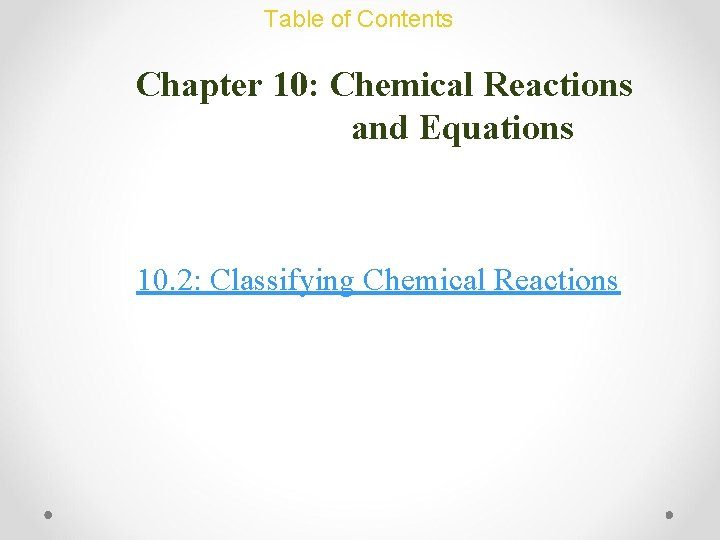Chemical Reactions and Equations: Basic Concepts Recognizing Chemical Reactions • When a substance undergoes a chemical change, it takes part in a chemical reaction. • After it reacts, it no longer has the same chemical identity.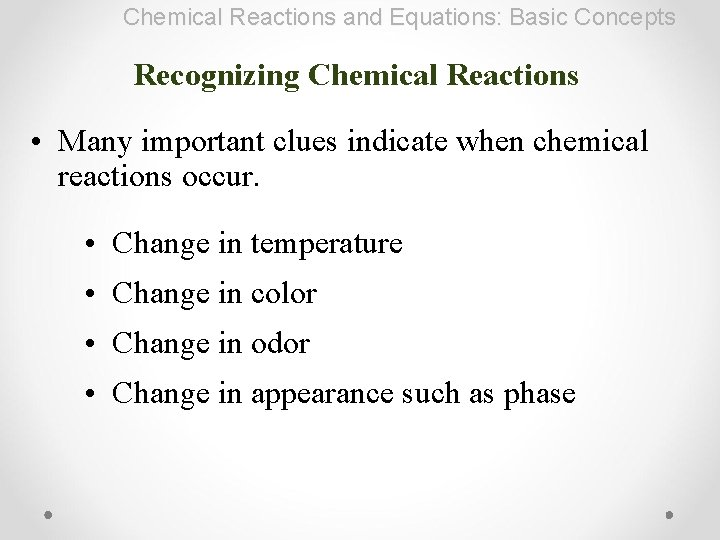Chemical Reactions and Equations: Basic Concepts Recognizing Chemical Reactions • Many important clues indicate when chemical reactions occur. • Change in temperature • Change in color • Change in odor • Change in appearance such as phase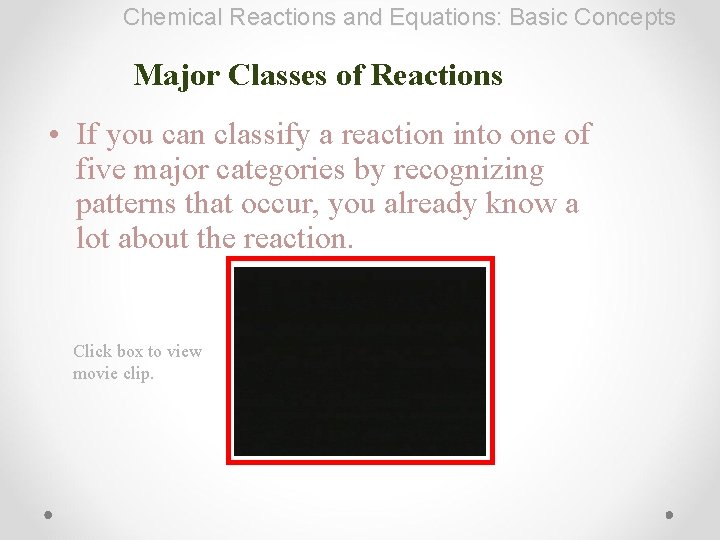Chemical Reactions and Equations: Basic Concepts Major Classes of Reactions • If you can classify a reaction into one of five major categories by recognizing patterns that occur, you already know a lot about the reaction. Click box to view movie clip.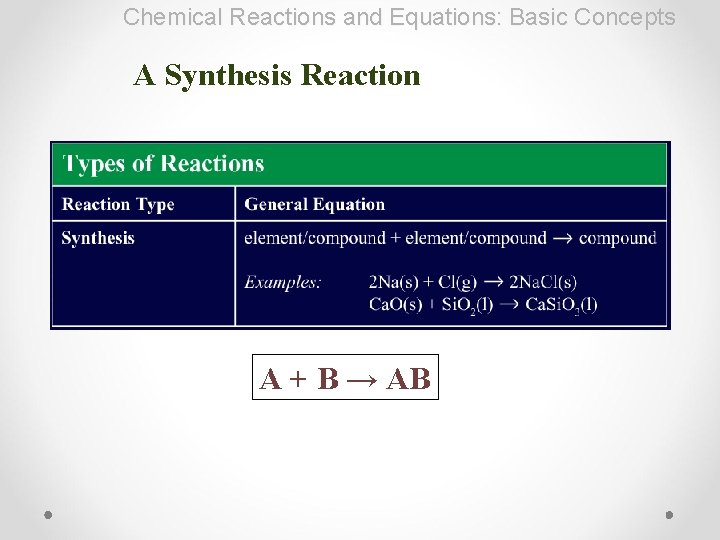Chemical Reactions and Equations: Basic Concepts A Synthesis Reaction A + B → ABChemical Reactions and Equations: Basic Concepts A Decomposition Reaction MCO 3(s) → MO(s) + CO 2(g) MOH(s) → MO(s) + H 2 O(g) MHCO 3(s) → MO(s) + H 2 O + CO 2(g) AB → A + BChemical Reactions and Equations: Basic Concepts Single Displacement A + BC → B + ACActivity Series For this type of reaction to occur, the “replacing” element MUST be more reactive than the “replaced” element. See table of reduction potentials. P. 288METALS Lithium Rubidium Potassium Calcium Sodium Magnesium Aluminum Manganese Zinc Iron Nickel Tin Lead Copper Silver Platinum Gold Most active P. 288 HALOGENS Fluorine Chlorine Bromine Iodine Least activeActivity Series F 2(g) + 2 Na. Br(aq) → 2 Na. F(aq) + Br 2(l) More active than Br Br 2(g) + 2 Na. F(aq) → NR Less active than F Most active HALOGENS Fluorine Chlorine Bromine Iodine Least active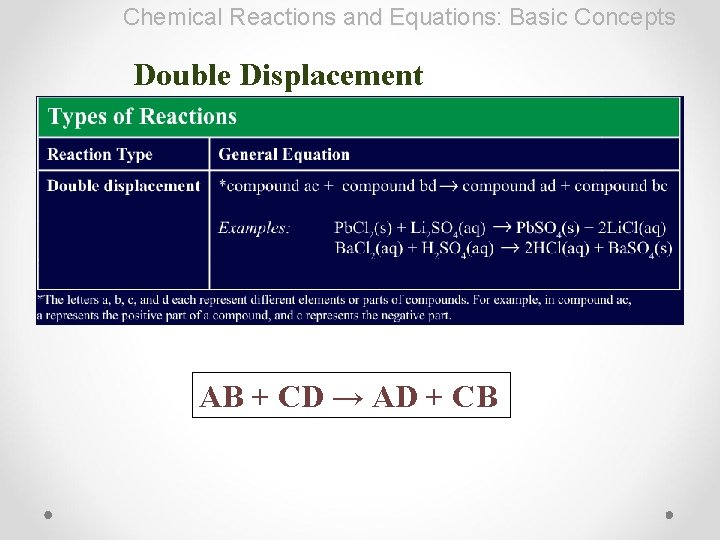Chemical Reactions and Equations: Basic Concepts Double Displacement AB + CD → AD + CBChemical Reactions and Equations: Basic Concepts Combustion Cx. Hy. Oz + O 2 → CO 2 + H 2 O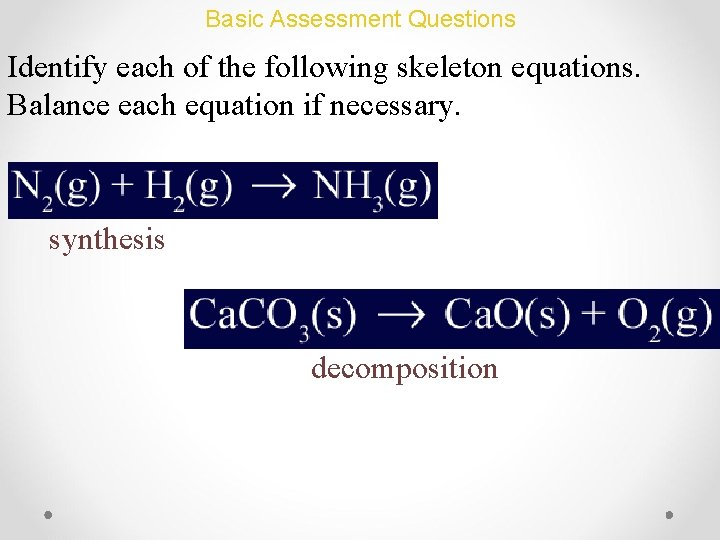Basic Assessment Questions Identify each of the following skeleton equations. Balance each equation if necessary. synthesis decomposition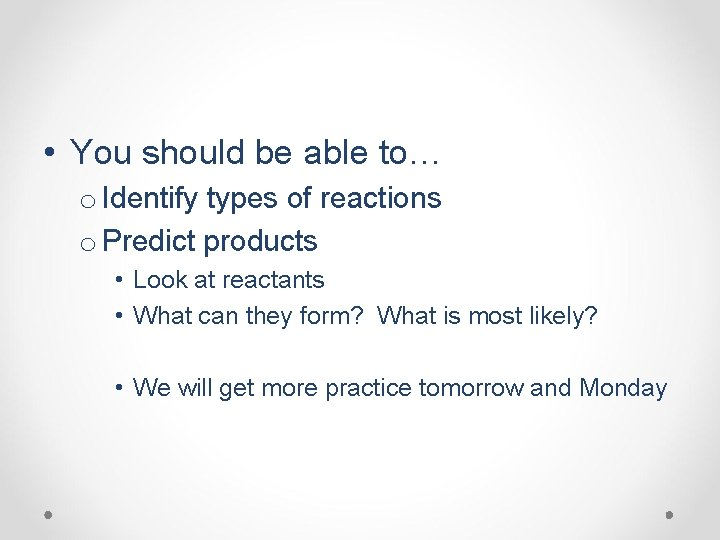• You should be able to… o Identify types of reactions o Predict products • Look at reactants • What can they form? What is most likely? • We will get more practice tomorrow and Monday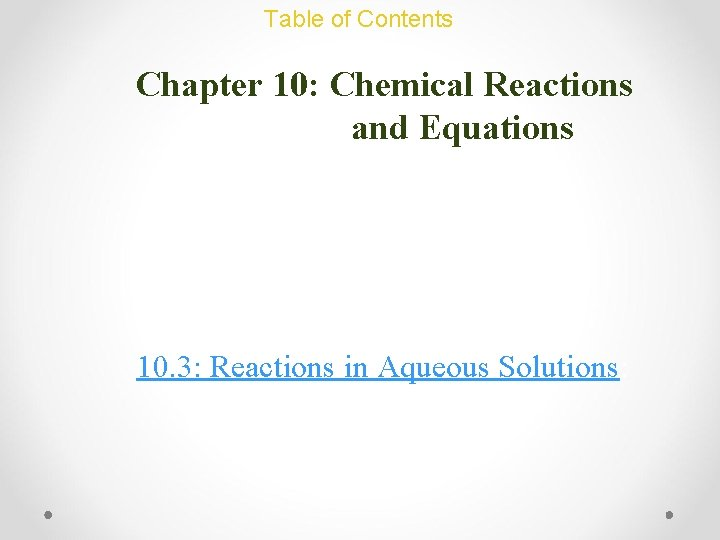Chemical Reactions and Equations: Additional Concepts REVIEW • With those at your table, please name and define the 5 classifications of reactionsChemical Reactions and Equations: Additional Concepts REVIEW • An iron bar reacts with oxygen to form rust. • What kind of reaction will take place? • What will the products be? H 2 O 4 Fe(s) + 3 O 2(g) → 2 Fe 2 O 3(s) synthesisChemical Reactions and Equations: Additional Concepts REVIEW • A copper wire is dipped into a silver nitrate solution. • What kind of reaction will take place? • What will the products be? Cu(s) + Ag. NO 3(aq) → Ag(s) + Cu. NO 3(aq) Single displacementChemical Reactions and Equations: Additional Concepts REVIEW • Methane gas (CH 4) from a Bunsen burner is lit on fire. • What kind of reaction will take place? • What will the products be? CH 4(g) + O 2(g) → CO 2(g) + H 2 O(g) CombustionChemical Reactions and Equations: Additional Concepts REVIEW • A Hydrogen balloon is lit on fire. • What kind of reaction will take place? • What will the products be? 2 H(g) + O 2(g) → 2 H 2 O(g) synthesis / combustionChemical Reactions and Equations: Additional Concepts Reactions in Aqueous Solutions • Solutions of calcium chloride and ammonium sulfate are mixed to form solid calcium sulfate. • What kind of reaction will take place? • What will the products be? Ca. Cl 2(aq) + (NH 4)2 SO 4(aq) → Ca. SO 4(s) + 2 NH 4 Cl(aq) Double displacement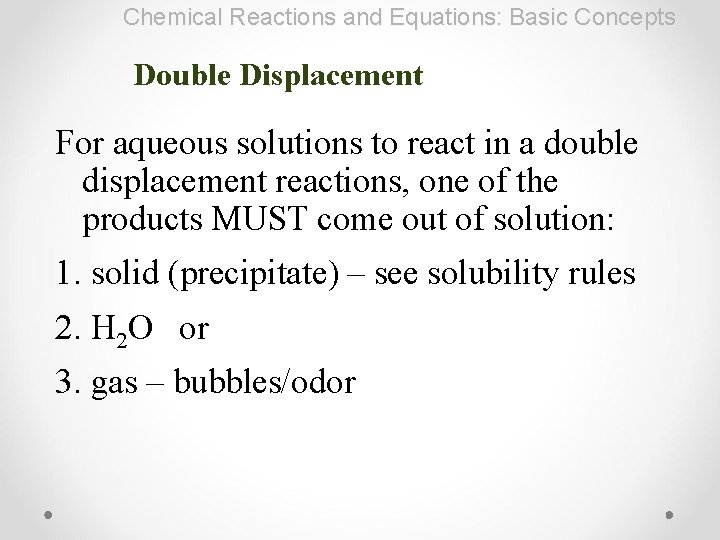Chemical Reactions and Equations: Basic Concepts Double Displacement For aqueous solutions to react in a double displacement reactions, one of the products MUST come out of solution: 1. solid (precipitate) – see solubility rules 2. H 2 O or 3. gas – bubbles/odorChemical Reactions and Equations: Additional Concepts Reactions in Aqueous Solutions precipitate • Check solubility on the back of the periodic table to see if a precipitate forms • If insoluble – it’s a precipitate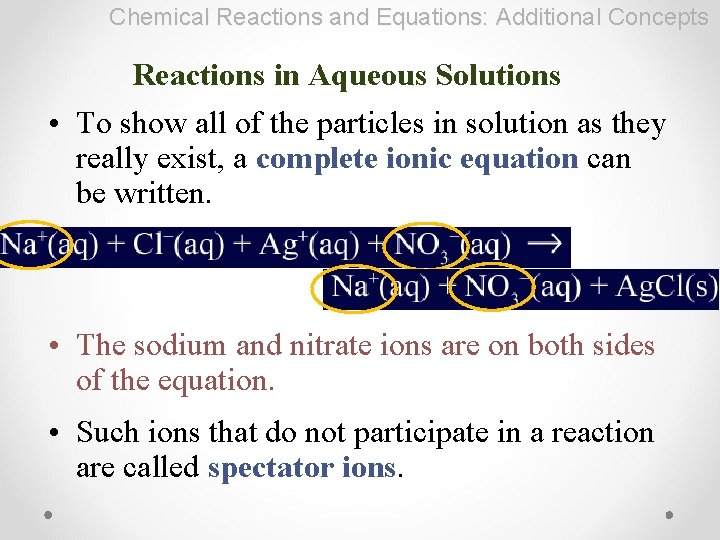Chemical Reactions and Equations: Additional Concepts Reactions in Aqueous Solutions • To show all of the particles in solution as they really exist, a complete ionic equation can be written. • The sodium and nitrate ions are on both sides of the equation. • Such ions that do not participate in a reaction are called spectator ions.Chemical Reactions and Equations: Additional Concepts Reactions in Aqueous Solutions • An ionic equation that does not show spectator ions but only the particles that participate in a reaction is called a net ionic equation. • In net ionic equations, (s), (l), (g) are never cancelled out.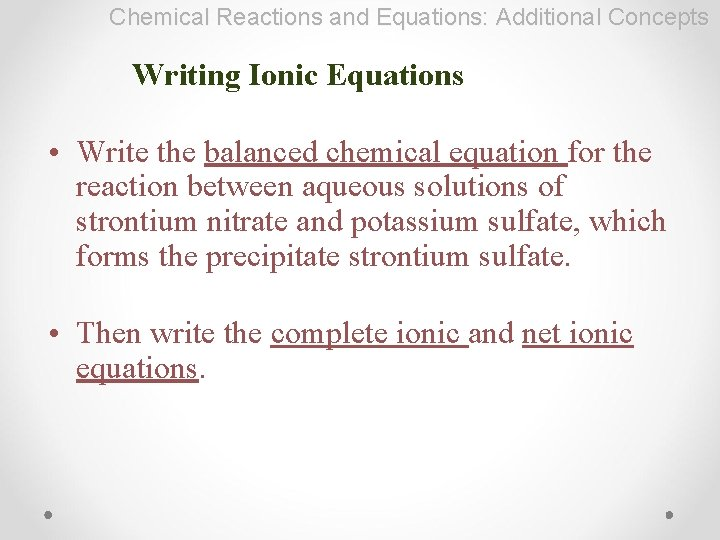Chemical Reactions and Equations: Additional Concepts Writing Ionic Equations • Write the balanced chemical equation for the reaction between aqueous solutions of strontium nitrate and potassium sulfate, which forms the precipitate strontium sulfate. • Then write the complete ionic and net ionic equations.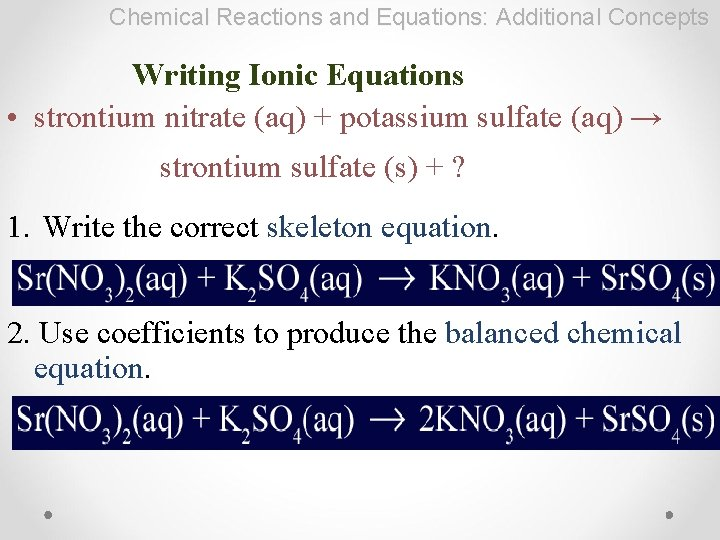Chemical Reactions and Equations: Additional Concepts Writing Ionic Equations • strontium nitrate (aq) + potassium sulfate (aq) → strontium sulfate (s) + ? 1. Write the correct skeleton equation. 2. Use coefficients to produce the balanced chemical equation.Chemical Reactions and Equations: Additional Concepts Writing Ionic Equations 3. Write the complete/total ionic equation. How to know when to split a compound: • Split only ionic (aq) compounds & strong acids (HCl, H 2 SO 4, HNO 3) • Leave molecules and weak acids alone! (HF, HCH 3 COO)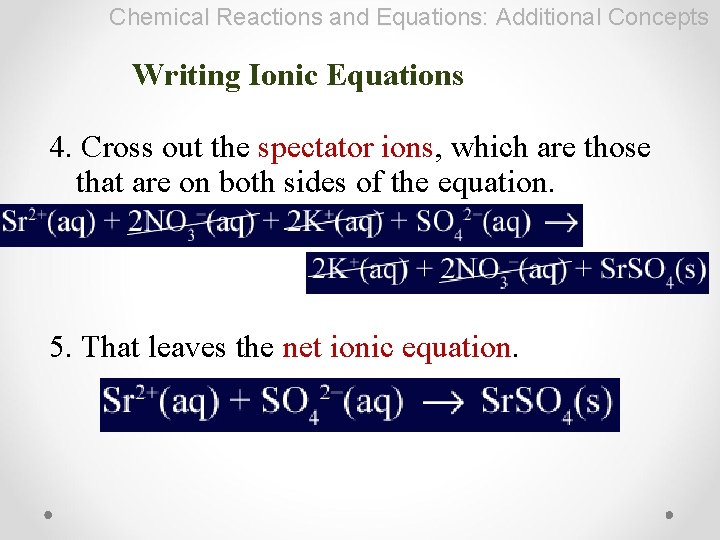Chemical Reactions and Equations: Additional Concepts Writing Ionic Equations 4. Cross out the spectator ions, which are those that are on both sides of the equation. 5. That leaves the net ionic equation.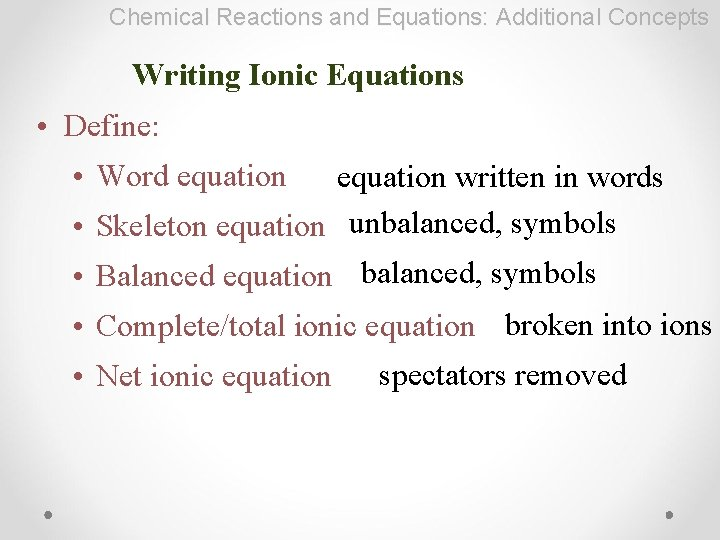Chemical Reactions and Equations: Additional Concepts Writing Ionic Equations • Define: • Word equation written in words • Skeleton equation unbalanced, symbols • Balanced equation balanced, symbols • Complete/total ionic equation broken into ions • Net ionic equation spectators removed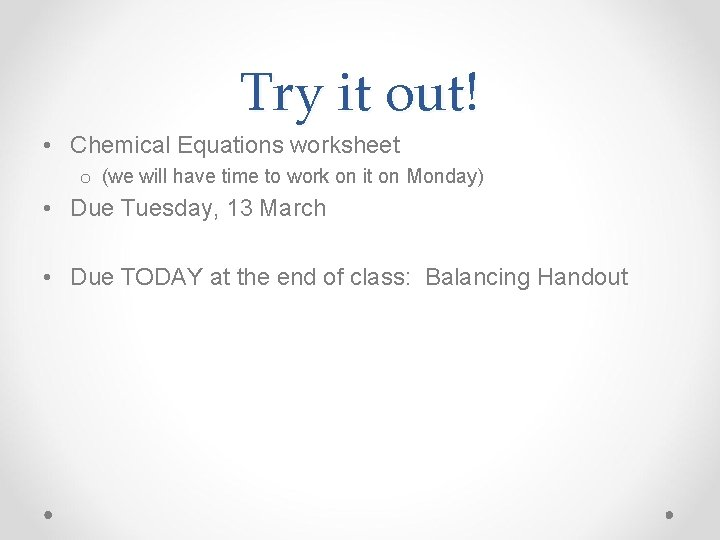Try it out! • Chemical Equations worksheet o (we will have time to work on it on Monday) • Due Tuesday, 13 March • Due TODAY at the end of class: Balancing Handout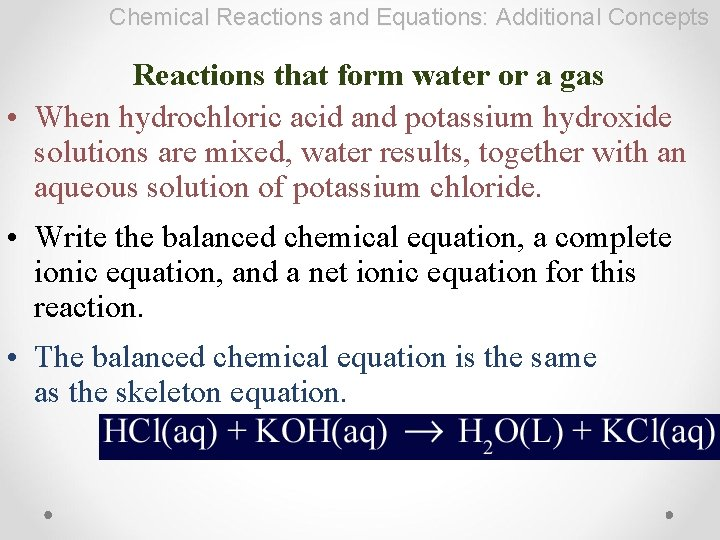Chemical Reactions and Equations: Additional Concepts Reactions that form water or a gas • When hydrochloric acid and potassium hydroxide solutions are mixed, water results, together with an aqueous solution of potassium chloride. • Write the balanced chemical equation, a complete ionic equation, and a net ionic equation for this reaction. • The balanced chemical equation is the same as the skeleton equation.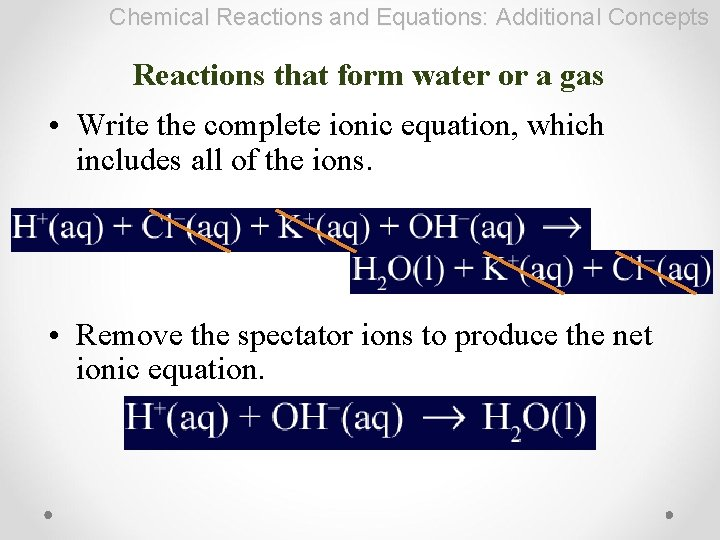Chemical Reactions and Equations: Additional Concepts Reactions that form water or a gas • Write the complete ionic equation, which includes all of the ions. • Remove the spectator ions to produce the net ionic equation.Additional Assessment Questions Question 1 Write a balanced chemical, complete ionic, and net ionic equations for the following reaction: Aqueous solutions of lead(II) nitrate and ammonium chloride are mixed, forming a precipitate.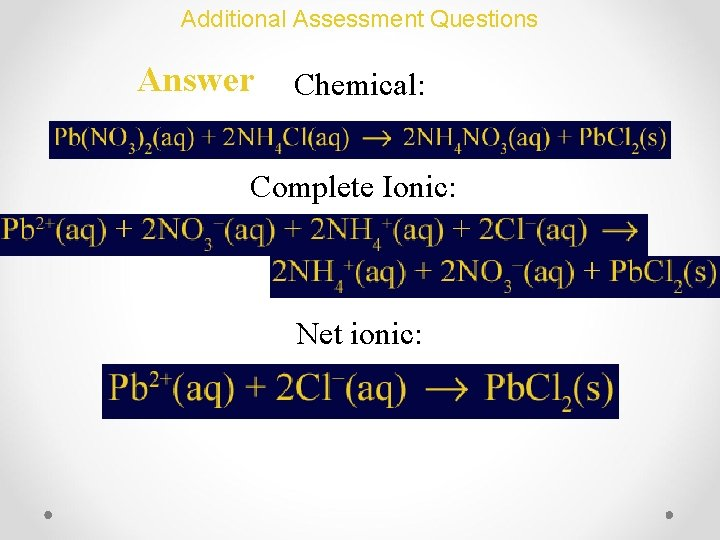Additional Assessment Questions Question 2 Write a balanced chemical, complete ionic, and net ionic equations for the reaction between the following substances, which produce water: nitric acid (HNO 3) and aqueous barium hydroxide.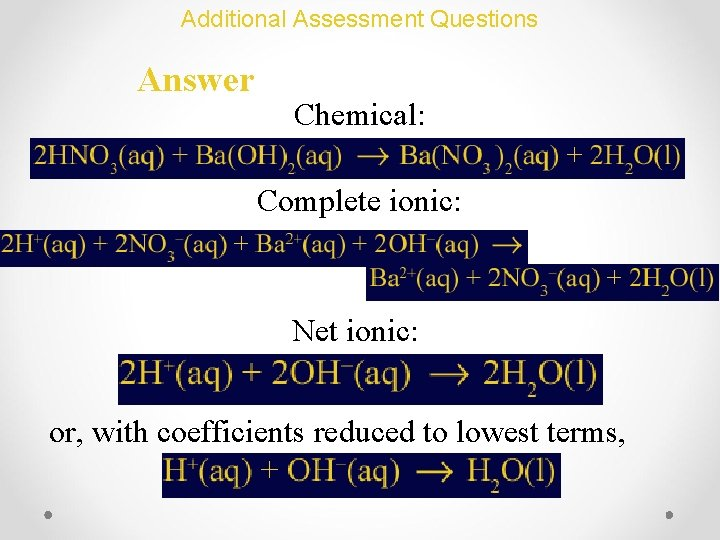Additional Assessment Questions Answer Chemical: Complete ionic: Net ionic: or, with coefficients reduced to lowest terms,Additional Assessment Questions Question 3 Write a balanced chemical, complete ionic, and net ionic equations for the reaction between the following substances, which produce a gas: hydrochloric acid and aqueous sodium cyanide, with production of a gas.